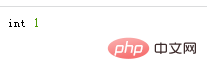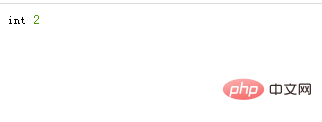php数组怎么查询元素位置php数组查询元素位置的方法：

<?php
\$array=array(2,3,4,1,5);
\$find=3;

//利用数组查找 找到所需要元素的索引位置
function find_by_array_search(\$array,\$find)
{
return array_search(\$find,\$array);
}
var_dump(find_by_array_search(\$array,\$find));
?>• 使用implode()将数组转为字符串

• 使用strpos() 函数查找字符串在另一字符串中第一次出现的位置。

<?php
\$array=array(2,3,4,1,5);
\$find=4;

//字符串查找
function find_by_string(\$array,\$find)
{
\$string=implode(\$array);
return strpos(\$string,\$find."");
}
var_dump(find_by_string(\$array,\$find));
?>全部评论我要评论

• 取消发布评论发送
• 1/1